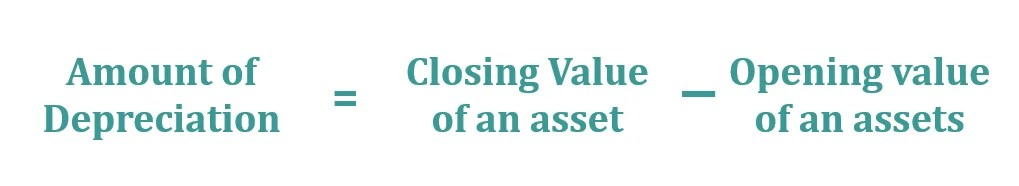# Revaluation Method of Depreciation | ExampleRevaluation Method of Depreciation Feature image

Under Revaluation Method of Depreciation, where we did not know the accurate life of an asset or where the life of an asset is uncertain and depreciation cannot be calculated with any other method then we will reevaluate an asset at the end of each financial year when balance sheet prepared. So, if any decrease in the book of an asset will be treated as the amount of depreciation or if any increase then it will be ignored.

## This method of depreciation is applied in the following cases: –

• Where we did not know the accurate life of an asset.
• Where the life of an asset is uncertain.
• Depreciation cannot be calculated with any other method.

### Example of Revaluation Method of Depreciation:

On 1st April 2018, The firm has loose tools worth Rs 21,000. These were valued at the end of each financial year shown as follows:-

1. 31/03/2019 – 18,900
2. 31/03/2020 – 17,700
3. 31/03/2021 – 16,550
4. 31/03/2022 – 15,250
5. 31/03/2023 – 14,150

Calculate the amount of depreciation.

Note: – To make a simple question we assume that no loose tool was broken in the whole period. “

#### Solution: –

Calculation of the amount of depreciation:-The following table shows the calculation of an amount of depreciation year by year:-

##### Amount of Depreciation
01-04-2018         21,000 31-03-2019      18,900                    2,100
01-04-2019         18,900 31-03-2020      17,700                    1,200
01-04-2020         17,700 31-03-2021      16,550                    1,150
01-04-2021         16,550 31-03-2022      15,250                    1,300
01-04-2022         15,250 31-03-2023      14,150                    1,100

Journal entries or ledger are the same with the following methods: